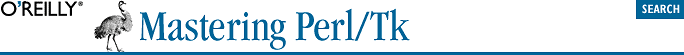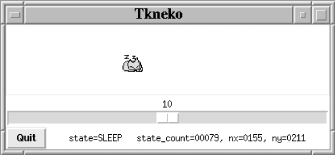home | O'Reilly's CD bookshelfs | FreeBSD | Linux | Cisco | Cisco Exam## 17.10. tkneko—Animating the Neko on a Canvas

To simulate motion, we display images of the neko at various positions on the Canvas at tenth of a second intervals (anything slower presents unacceptable flicker). The images we have to work with are shown in Figure 17-14. To make the neko run left, we repeatedly display the left1.ppm and left2.ppm images. (If the images display at the same Canvas coordinate, the neko runs in place. We might as well have used Tk::Animation if we wanted that effect.)

The neko's actions are state driven.

 The "togi" states are from the original Japanese code. We don't pretend to know what they mean, although they are the "scratching the wall" states.

There are in fact five distinct states: the neko is either waking, moving, stopping, scratching, or sleeping. The tkneko states are encoded in a Perl hash with compiled (for efficiency) regular expressions as keys and code references (the state processors) as values:

```%states = (
qr/AWAKE/                   => \&do_awake,
qr/UP|UPRIGHT|RIGHT|DWRIGHT|DOWN|DWLEFT|LEFT|UPLEFT/ => \&do_move,
qr/STOP/                    => \&do_stop,
qr/UTOGI|RTOGI|DTOGI|LTOGI/ => \&do_togi,
qr/SLEEP/                   => \&do_sleep,
);           # neko state table```

The states that are dependent on the neko's direction (but otherwise equivalent) are further divided into substates, described by a regular expression with alternatives.

go_neko, the animation main loop, is activated by a repeating 100 millisecond timer event. The subroutine's job is simply to call a subroutine based on the animation's current state, \$state. The subroutine in turn selects an appropriate PPM image and displays it on the canvas.

```\$mw->repeat(100 => \&go_neko);

sub go_neko {

\$state_count++;              # current state's cycle count
\$where = sprintf("state=%-7s state_count=%05d, nx=%04d, ny=%04d",
\$state, \$state_count, \$nx, \$ny);

STATES:
foreach my \$regex (keys %states) {
next STATES unless \$state =~ /^(\$regex)\$/;
&{\$states{\$regex}}(\$1);
return;
}

} # end go_neko```
```foreach my \$pfn ( <\$image_base/*.ppm> ) {
my \$bpfn = basename \$pfn;
\$pixmaps{\$bpfn} = \$canvas->createImage(-1000, -1000,
-image => \$canvas->Photo(-file => \$pfn));
}  ```#### Hidden Canvas Items

After this chapter was written, Tk 800.018 introduced the -state option for individual Canvas items, whose value can be normal, disabled, or hidden. We can take advantage of this and instead of moving an image offscreen, simply mark it as hidden:

`\$canvas->itemconfigure(\$pixmaps{\$pxid}, -state => 'hidden');`

This is the preferred solution, because to be sure that the image we move is offscreen, we need to factor in the current -scrollregion:

```@scrollregion = @{\$canvas->cget(-scrollregion) };
\$canvas->coords(\$pixmaps{\$pxid},
\$scrollregion - 1000, \$scrollregion - 1000);```

The -scrollregion option is a reference to an array of two canvas coordinates (four items): the top-left corner and bottom-right corner of a bounding box describing the maximum extents that one may scroll the canvas. For instance:

`\$canvas->configure(-scrollregion => [-1100, -1100, 400, 400]);`

defines a square canvas 1500 pixels per side that in theory can be scrolled up-and-left so that our "hidden" Canvas items become visible. To be really sure the image is hidden, we should substitute the image's width and height for the constants 1000.

 Once again, for Tk Version 800.018 and newer it's preferable to set an image's state to hidden to make it disappear, and normal to make it visible.

```sub frame {
\$canvas->coords(\$pixmaps{\$pix}, -1000, -1000);
\$pix = "\$_.ppm";
\$canvas->coords(\$pixmaps{\$pix}, \$nx, \$ny);
}```

So do_move might make a call such as this to make the neko run left:

`frame 'left' . ((\$state_count % 2) + 1);`

Of course, in the actual program, the direction isn't a hardcoded string but the back-reference \$1 from the state table's regular expression match.

To make the neko follow the cursor, we use Tk's pointerxy command to get the cursor's coordinates, compute a heading from the neko to the cursor, and then map that value to a new state. \$r2d is the radian-to-degree conversion factor, and \$h is the new heading, in degrees.

```(\$x, \$y) = \$canvas->pointerxy;

my \$h = int( \$r2d * atan2( (\$y - \$ny), (\$x - \$nx) ) ) % 360;
my(\$degrees, \$dir);

foreach (
[[ 22.5,  67.5], 'DWRIGHT'],
[[ 67.5, 112.5], 'DOWN'],
[[112.5, 157.5], 'DWLEFT'],
[[157.5, 202.5], 'LEFT'],
[[202.5, 247.5], 'UPLEFT'],
[[247.5, 292.5], 'UP'],
[[292.5, 337.5], 'UPRIGHT'],
[[337.5,  22.5], 'RIGHT'],
) {
(\$degrees, \$dir) = (\$_->, \$_->);
last if \$h >= \$degrees-> and \$h < \$degrees->;
} # forend

set_state \$dir;```